# Self Weight

## Function

• Enter the self-weight of elements included in the model as applied loads, or modify or delete previously entered self-weight.
• midas CIVIL uses the volume and density of each element to automatically calculate the self-weight included in the analysis model.
• The computed self-weight can be considered in static analysis for the global coordinate system in each direction (X, Y, and Z) as a body force using the Self Weight feature.
• In addition, "Structure Type" determines if the mass effect due to the self-weight is to be considered in the dynamic analysis or in the calculation of the equivalent static seismic load.
• The methods for considering the self-weight for different element types are as follows :

1. Truss, tension-only, compression-only and beam elements

The self-weight of a truss, tension-only, compression-only or prismatic beam element is calculated by the cross sectional area and the density entered in "Section" and "Material", multiplied by the element length uniformly distributed over the entire length of the element.

For a beam element defined as a SRC section (Steel and Reinforced Concrete Composite Section), (refer to the "SRC" tab in Section) the weight is considered by separately calculating concrete and steel weights.

If a beam element is defined as Tapered Section (refer to the "Tapered" tab in "Section"), it is assumed that the self-weight varies linearly from one end to the opposite end.

When considering the effects of Panel Zone Effects, the self-weight of beam elements classified as column members is considered based on the length between the two end points without considering the panel zone length. On the other hand, for beam elements classified as girder members (horizontal members connected to columns), the self-weight is calculated using the length between the two end points excluding the panel zone length and the distance between the two ends.

If an end offset distance is introduced in a prismatic beam element by "Beam End Offsets", it is assumed that the self-weight is uniformly distributed over the entire length between both nodes, irrespective of the offset.

In the case of a tapered section, it is assumed that the average self-weight is also uniformly distributed over the panel zone offset distances.

2. Plane stress, plate, plane strain and axisymmetric elements

The self-weight of a plane stress, plate, plane strain or axisymmetric element is entered at the connection nodes as concentrated loads obtained by multiplying the element's cross sectional area, thickness and density, considering the area ratio.

The thickness data entered in "Thickness" (in-plane thickness in the case of plate elements) are used for plane stress and plate elements. The unit width (1.0) and unit angle (1.0 radian) are used for plane strain and axisymmetric elements respectively.

3. Solid elements

The self-weight of a solid element is entered at each connection node as concentrated loads obtained by multiplying the volume by the density considering the volume ratio.

## Call

From main menu, select [Load] tab > [Type : Static Loads] > [Structure Loads / Masses] group >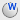[Self Weight]

## Input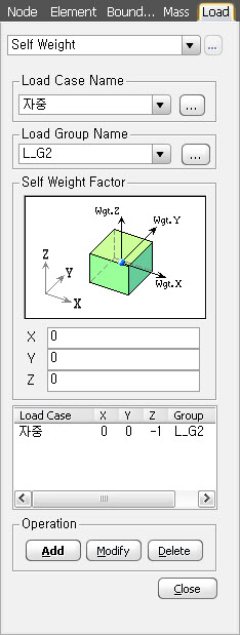Self Weight dialog box

Select the desire Load Group that will include the entered Self Weight load data. Select "Defualt", if a Group assignment is unnecessary. Click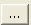to the right to add, modify or delete Load Groups.

Select the desire Load Group that will include the entered Self Weight load data. Select "Defualt", if a Group assignment is unnecessary. Clickto the right to add, modify or delete Load Groups.

### Self Weight Factor

X : Self-weight factor in the GCS X-direction

Y : Self-weight factor in the GCS Y-direction

Z : Self-weight factor in the GCS Z-direction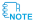If the entered in-plane and out-of-plane thicknesses are different, in-plane thickness is used for calculating the Self weight. However, when in-plane thickness is '0' out-of-plane thickness will be used.For Self weight of Stiffened Plate, the program uses greater of the in-plane thicknesses - yz section and xz section. However, when in-plane thickness is '0' the greater of out-of-plane thicknesses will be used.When "Change with Tendon" is selected in the Construction Stage Analysis Control Data, tendon weight will not be included in the structure weight but will be reflected in the section stiffness. Before grouting, the self-weight is calculated based on the net area of concrete section (area excluding sheathing), whereas after grouting the self-weight is based on the total area of concrete section.

### Operation

Enter the following items and click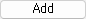.

Modify

Select the relevant case from the self-weight list at the bottom of the dialog box, modify the entry field at the top and click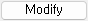.

Delete

Select the relevant load case from the self-weight list at the bottom of the dialog box and click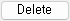.

0